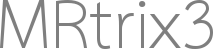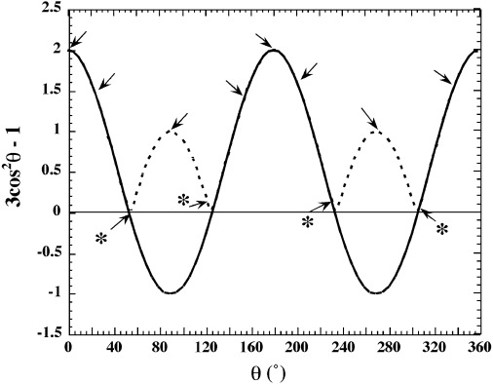# Fiber Orientation with respect to Main Magnetic Field and Magic Angle of Knee Joint

Dear MRtrix3 Community,

I would like to post one topic here. I calculated the angle with respect to the main magnetic field from fibre orientation data. The screenshot is attached. The image is an Anterior Cruciate Ligament (ACL) of an MRI knee joint. The code is as follows.

``````mrconvert -coord 3 2 fiber.nii zcomponent.nii
mrcalc zcomponent.nii -acos angle.nii
mrcalc angle.nii pi -divide 180 -multiply angledeg.nii
mrconvert -coord 3 0 -axes 0,1,2 angledeg.nii.gz angledeg3d.nii.gz
``````Now, I would like to calculate the magic angle effect. For this, I would like to create a graph like this.Additionally, I also have a T2* image.

Thank you very much.

-Suren

Hello MRtrix3 Experts,

I calculated fiber orientation in the direction of main magnetic field according to the above codes. I would like to know whether it is possible to calculate fiber orientation at some angle from the main magnetic field such as 10 degrees or any other angle.

Thank you very much.

Suren

Hi Suren,

Your original question is a little hard to follow. The graph that you show is of a parametric relationship, which is void of any image-based information. By pooling data across many voxels, where for each voxel you have both an angle with respect to the main magnetic field and , you would be able to export those data to some other software to produce a scatter plot with angle on the x-axis and on the y-axis. But it’s not immediately clear what you intend that metric of interest to be (though I think the logic holds for whatever that metric may be). Regardless, since you need to get those raw image values into some other software to deal with plotting, I think ultipmately what you’re looking for is the `mrdump` command.

I would like to know whether it is possible to calculate fiber orientation at some angle from the main magnetic field such as 10 degrees or any other angle.

This is also unclear to me. For some fixed angle (e.g. 10 degrees), there’s an infinite set of directions on the sphere that are 10 degrees away from the z-axis, forming a circle on S2. But these cannot be fibre orientations since there’s no image data involved.

One possibility is that you are wanting to find a set of voxels where the estimated fibre orientation is approximately 10 degrees away from the z-axis? If that’s the case, it’s just a matter of breaking down the computation:
`mrcalc angle.mif 10.0 -sub -abs - | \`
`mrthreshold - -bottom N voxels_10degrees.mif`
The first line calculates, for each voxel, the deviation of that fibre orientation from the circle on S2 that is 10 degrees away from the z-axis; the second line selects those N voxels for which this angle is minimal.

If this isn’t what you’re looking for you might need to try an alternative phrasing of the question.

Cheers
Rob

1 Like